# 图像倾斜 数字图像处理

## python 图像倾斜校正

2019-07-30 16:31:25 Wuli_GDa 阅读数 1565
• ###### matlab数字验证码识别

matlab数字验证码识别 </p>

3课时 8分钟 589人学习 刘昱显
免费试看

• 边缘检测
边缘检测容易受到噪声影响，一般第一步都是用5*5的高斯滤波器去除噪声
``````#!/usr/bin/env python
# -*- coding:utf-8 -*-
import numpy as np
import cv2

img = cv2.GaussianBlur(img,(3,3),0)#高斯模糊  去噪 以免影响边缘检测
gray = cv2.cvtColor(img,cv2.COLOR_BGR2GRAY)

edges = cv2.Canny(gray,50,250,apertureSize = 3)#边缘检测
cv2.imwrite("canny.jpg", edges)
``````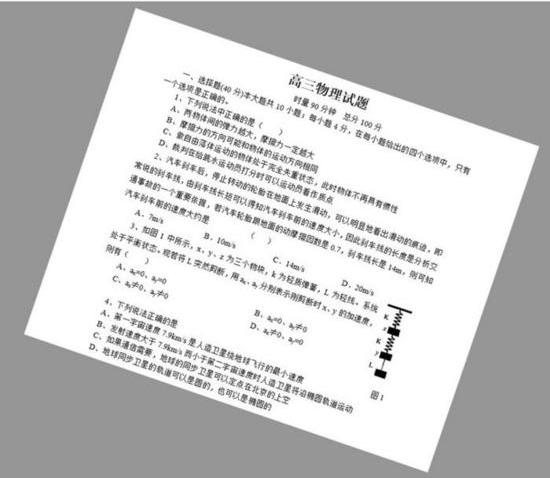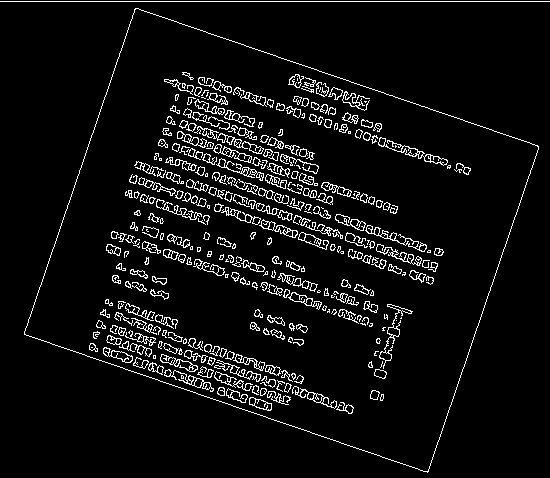cv2.Canny()函数的函数原型为：

``````edge = cv2.Canny(image, threshold1, threshold2[, edges[, apertureSize[, L2gradient ]]])
``````
• 第一个参数是输入图像，必须是单通道的灰度图；
• 第二个和第三个参数是minVal和maxVal；
• apertureSize 为卷积核大小，默认为3；
（卷积核：卷积核具有的一个属性就是局部性。即它只关注局部特征，局部的程度取决于卷积核的大小。比如用 Sobel 算子进行边缘检测，本质就是比较图像邻近像素的相似性。）

python Canny参考资料

## 车牌图像倾斜校正算法的MATLAB实现

2018-06-19 23:43:06 qq_15971883 阅读数 16027
• ###### matlab数字验证码识别

matlab数字验证码识别 </p>

3课时 8分钟 589人学习 刘昱显
免费试看

1. 基于Hough变换的车牌图像倾斜校正算法

利用Hough变换检测车牌的边框，确定边框直线的倾斜角度，根据倾斜角度旋转，获得校正后的图像。具体步骤如下：

• 图像预处理。读取图像，转换为灰度图像，去除离散噪声点。
• 利用边缘检测，对图像中的水平线进行强化处理。
• 基于Hough变换检测车牌图像的边框，获取倾斜角度。
• 根据倾斜角度，对车牌图像进行倾斜校正。
``````% 利用hough变换实现车牌图像的倾斜校正
close all; clear all; clc;
figure;
subplot(131), imshow(I);
I1 = rgb2gray(I);  % 将原始图像转换为灰度图像
I2 = wiener2(I1, [5, 5]);  % 对灰度图像进行维纳滤波
I3 = edge(I2, 'canny');  % 边缘检测
subplot(132), imshow(I3);
[m, n] = size(I3);  % compute the size of the image
rho = round(sqrt(m^2 + n^2)); % 获取ρ的最大值，此处rho=282
theta = 180; % 获取θ的最大值
r = zeros(rho, theta);  % 产生初值为0的计数矩阵
for i = 1 : m
for j = 1 : n
if I3(i,j) == 1  % I3是边缘检测得到的图像
for k = 1 : theta
ru = round(abs(i*cosd(k) + j*sind(k)));
r(ru+1, k) = r(ru+1, k) + 1; % 对矩阵计数
end
end
end
end
r_max = r(1,1);
for i = 1 : rho
for j = 1 : theta
if r(i,j) > r_max
r_max = r(i,j);
c = j; % 把矩阵元素最大值所对应的列坐标送给c
end
end
end
if c <= 90
rot_theta = -c;  % 确定旋转角度
else
rot_theta = 180 - c;
end
I4 = imrotate(I1, rot_theta, 'crop');  % 对图像进行旋转，校正图像
subplot(133), imshow(I4);
set(0, 'defaultFigurePosition', [100, 100, 1200, 450]); % 修改图像位置的默认设置
set(0, 'defaultFigureColor', [1, 1, 1]); % 修改图像背景颜色的设置``````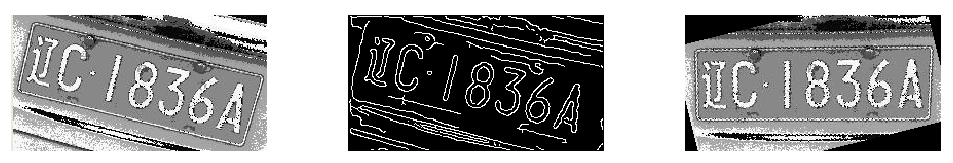将车牌图像朝各个方向投影，进而通过分析各方向的投影特性确定车牌的倾斜角度。具体的实现步骤如下：

• 图像预处理。读取图像，转换为灰度图，去除离散噪声点。
• 利用边缘检测，对图像中水平线进行强化处理。
• 根据倾斜角度，对车牌图像进行倾斜校正。
``````close all; clear all; clc;
subplot(131), imshow(I);
I1 = rgb2gray(I);
I2 = wiener2(I1, [5, 5]);
I3 = edge(I2, 'canny');
subplot(132), imshow(I3);
theta = 0 : 179;
[m, n] = size(r);
c = 1;
for i = 1 : m
for j = 1 : n
if r(1,1) < r(i,j)
r(1,1) = r(i,j);
c = j;
end
end
end
rot_theta = 90 - c;
I5 = imrotate(I, rot_theta, 'crop');
subplot(133), imshow(I5); ``````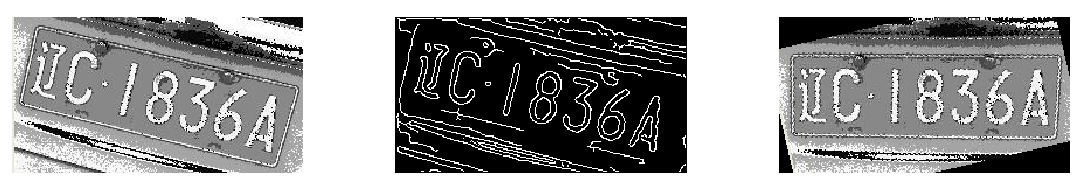## 数字图像处理 知识点

2019-01-13 23:50:27 weixin_37303854 阅读数 441
• ###### matlab数字验证码识别

matlab数字验证码识别 </p>

3课时 8分钟 589人学习 刘昱显
免费试看

## 基础概念

• 数字图像：图像可定义为一个二维函数f(x,y),幅值f为图像的强度（灰度）。当x,y,f是有限的离散数值时，该图像为数字图像。
• 数字图像处理：借助于数字计算机来处理数字图像。
• 亮度函数、入射分量、反射分量• 马赫带效应• 同时对比度• 亮度恒定：当物体对背景的亮度、对比度保持一致时，即使物体和背景的亮度在很大的范围里变化，人眼对亮度的感觉仍保持不变。
• 空间频率特征：空间频率是指视像空间变化的快慢。清晰明快的画面，意味这有大量的高频成分。模糊图像只有低频空间成分。
• 图像的取样与量化：数字化坐标轴称为取样，数字化幅度值称为量化。

## 图像属性

• 动态范围：有时灰度级取值范围称为图像的动态范围。
• 灰度级：灰度级的典型的取值是2的整数次幂。通常假设离散灰度级是等间隔的并且是区间[0,L-1]内的整数 。
• kbit图像：当一副图像有2k灰度级时，通常称该图像是K比特图像
• 图像分辨率：实际上指对原始图像的采样分辨率。单位“象素点/单位长度”
• 采样分辨率：单位长度上所包含的采样数
• 灰度分辨率：灰度级中可分辨的最小变化（8比特的灰度分辨率图像有256个灰度级）
• 图像深度：在位图图像中，表示各象素点亮度或色彩信息的二进制位数。
• CMYK RGB HSI (HSV,HSB,HSL)

## 基础计算

• 点运算：是点对点的对灰度值进行计算• 代数运算：图像点对点的代数运算，不是矩阵的运算• 几何运算：涉及图像的转动、扭曲、倾斜、拉伸，空间点位置的变化、灰度值的变化
• 最邻近插值：最近的输入像素的灰度值
• 双线性插值线性插值：令f（x,y）为两个变量的函数，其在单位正方形顶点的值已知。假设我们希望通过插值得到正方形内任意点的f(x,y)值。我们可由如下双曲线方程：f(x,y)=ax+by+cxy+d
• 灰度变换：将一个灰度区间映射到另一个灰度区间的变换称为灰度变换。
• 直方图 累积直方图 连续图像幅度的密度函数，幅度分布函数
• 灰度直方图：灰度直方图是灰度级的函数，它表示图像中具有某种灰度级的像素的个数，反映了图像中某种灰度出现的频率。
• 线性系统： 叠加性+齐次性 ay1+by2=T[ax1+bx2]
• 移不变系统：是指如果输入序列进行移位，则输出序列进行相应的移位。
• 线性移不变系统：同时具有线性性和移不变性。
• 卷积## 傅立叶

• 傅立叶变换对(公式)• 傅立叶振幅谱 傅立叶相位谱 傅立叶能量谱## 频域滤波• （理想）低通滤波
具有振铃现象• 巴特沃思低通滤波BLPF模糊程度减少但尾部含有较多的高频，对噪声的平滑效果不如ILPF。
• 指数低通滤波器梯形低通滤波器
• 高通滤波
• 同态滤波取对数、傅立叶变换、乘上高/低通过滤器、傅立叶逆变换、求指数

## 图像增强技术 图像复原技术

• 退化：图像的质量变坏叫做退化。退化的形式有图像模糊、图像有干扰等
• 均值滤波 中值滤波 最大值滤波 最小值滤波 中点滤波((最大+最小)/2)
• 自适应中值滤波## 彩色图像

• 安全色216种
• RGB/CMYK
• HSI：色调、饱和度、亮度• 伪彩色：伪彩色处理是指将黑白图像转化为彩色图像，或者是将单色图像变换成给定彩色分布的图像。主要有密度分层法、灰度级-彩色变换法、频域滤波法

• 腐蚀、膨胀
• 开操作：先腐蚀，再膨胀
• 闭操作：先膨胀，再腐蚀
• 边缘提取填充

## 图像分割

• Roberts算子• Prewitt算子• Sobel算子• 拉普拉斯算子• 域值法：全局门限，局域门限，动态\自适应门限
• 全局域值自动分割
对于有明显双峰得得直方图可以通过程序,然计算机自动实现.算法:
1.选择一个初始化得估计域值T.
2.用T分割图像,生成两组数据,G1,G2;
3.求两组数据的平均灰度值u1,u2
4.计算新门限值:T=(u1+u2)/2
5.重复2到4,直到迭代所得到的T值之差小于指点的参数T0.
• 区域分裂与合并
• 分水岭算法

## 图像表示与描述

• 链码 多边形近似 边界分段 标记图
• 一阶差分码 形状数
• 傅立叶描述子
• 统计矩## 计算

• 直方图均衡化  直方图规定化
• 形态学 操作（腐蚀、膨胀、开、闭、填充……）
• 图像表示：链码 一阶差分码 形状数 灰度共生矩

## 数字图像处理>>>1.3数字图像处理系统

2020-02-26 22:07:48 richowe 阅读数 167
• ###### matlab数字验证码识别

matlab数字验证码识别 </p>

3课时 8分钟 589人学习 刘昱显
免费试看
##### 数字图像处理系统

• 数字图像输入模块：获取图像，通过数字设备将要处理的连续图像转换为计算机处理的数字图像
• 数字图像存储模块：作为存储单元存储数字图像数据
• 数字图像输出模块：将处理前后的数字图像显示出来或者永久保存
• 数字图像通信模块：对图像进行传输和通信
• 数字图像处理和分析模块：通用图像处理、专用图像处理、图像处理芯片，一般包括处理算法、实现软件和计算机组成
如下图所示：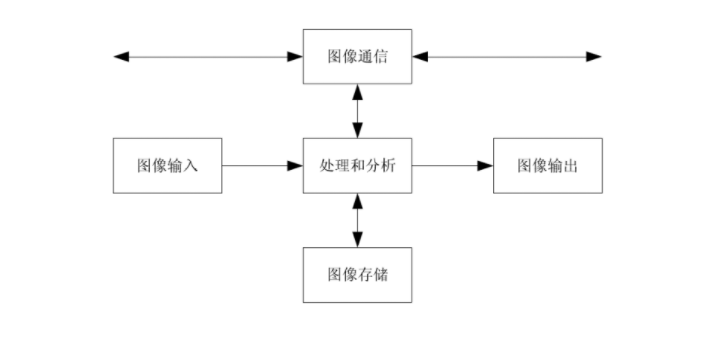##### 图像基础
• 图像表达：建模，图像采样、数字化
• 图像和视觉基础
• 图像变化：提高图像质量
##### 图像处理
• 图像增强：改善图像质量
• 图像几何处理：平移、缩放、旋转、扭曲
• 图像复原：去噪声、去模糊
• 图像重建：重建原始图像
• 图像编码压缩：减少存储量和传输量
• 图像分割：图像区域分割和理解目标表达和描述

## 图像处理 倾斜检测

2013-02-20 16:36:25 ace12304568 阅读数 3795
• ###### matlab数字验证码识别

matlab数字验证码识别 </p>

3课时 8分钟 589人学习 刘昱显
免费试看

在ocr（数字图像文本识别）过程中，由于图像的不可控性，总会存在一定角度的倾斜。倾斜角度要满足一定范围：

θ≤d/L         （θ即倾斜角度，d为文本行距，L为文本行长）

若超出这个范围，则可能将下（上）一行的文字拼接到本行，替代原有文字，产生误断。

通常来讲：θ应在2度以内。

为了避免或者是纠正这种问题，就需要对图像进行预处理，进行倾斜检测：

有如下假设：搜索图像都已进行了二值化处理（非黑即白）（常用方法：中值阈值，最佳阈值，otu算法）。图像大小为M*N，图像只是倾斜，并未上下翻转。

直线在想素质上的特点：扫描图像中一条直线方程上的坐标点，会发现黑点最多（N<Max（M，N））。

方法1：投影法。

思想是：如图所示，扫描有限条直线（m条），统计每条直线上法线方向黑点最多（最少）的一斜行。在所有最值中，最大的那行所对应的斜率mx/n的绝对值即倾斜角度的正切值。实现方法：输入int[][] pixels;

输出int[][]  A;

步骤: 1初始化参数int a=n, b=0, count=0, max=0,c=0;

直线方程：y=ax/b；法线方程y=bx/a+c

2     for(;b<m;b++){

max=0;

for(int i=0;i<n;i++){

//统计对应斜率（a，b）时，过点（i*b/a,i）的法线上的黑点数;

count=0;  c=(a*a-b*b)*i/a*a

for(int j=0;j<m;i++)

if(pixels[j][b/a*j+c]==1)count++;

if(count>max){

max=count;

A[b]=i;//记录每种斜率上黑点最多的斜行，和最大值；

A[b]=max;

}

}

}

3接下来是对数组A的分析了。这个数组保存了图像各个方向上最长直线的斜率和位置。

选择合理的其中一个作为倾斜角度，即可。也可简单的只去最大值；因为文本图像通常没有很

多的噪声，不会出现大的色块。其实依照数组你甚至可以重绘所有被检测到的直线。

方法2：hough变换：

原理：

图像中任意一条直线的方程可写为Ax+By=C；做归一化处理可得方程Ax+By=1；那么一条直线将唯一的被数对（A，B）确定。

过任意一点（a，b）的直线方程为Ma+Nb=1；若p1(a,b),p2(c,d)共线（不是几何意义的共线，而是有条线，两点均在此线上）Ax+By=1可得：M1a+N1b=1与M2c+N2d=1交于(A，B)点.

如图所示：0.初始条件：int[][] AB;

1.i=0->m;j=0->n

if(pixels[i][j]==1)

a=0->max；b=0->max

if(ai+bj==1)

AB[i][j]++;

2.寻找AB中的最大值，其对应坐标（i，j）；就是所求倾斜角的-i/j就是倾斜角的正切值。

实现：

上述原理中有一处不好处理就是AB的取值范围，不好确定。

做如下改进即可，原图像中直线的方程设为：

p=xcosa+ysina；(a为图像中直线倾斜角度的余角，p为原点到直线的距离)

则A->p/sina  b->p/cosa  0<a<180  0<p<sqrt(m*m+n*n);

即做了一个十字坐标系xoy到极坐标系poa的转换。

PS：由此可以看到直线检测在图像处理中是如何运作的。是跟曲线方程特征而决定的。同理其他曲线，也可根据曲线方程的特点有hough变换得到。投影法就略显困难。因此hough变换被广泛用于图像中图形识别过程中。